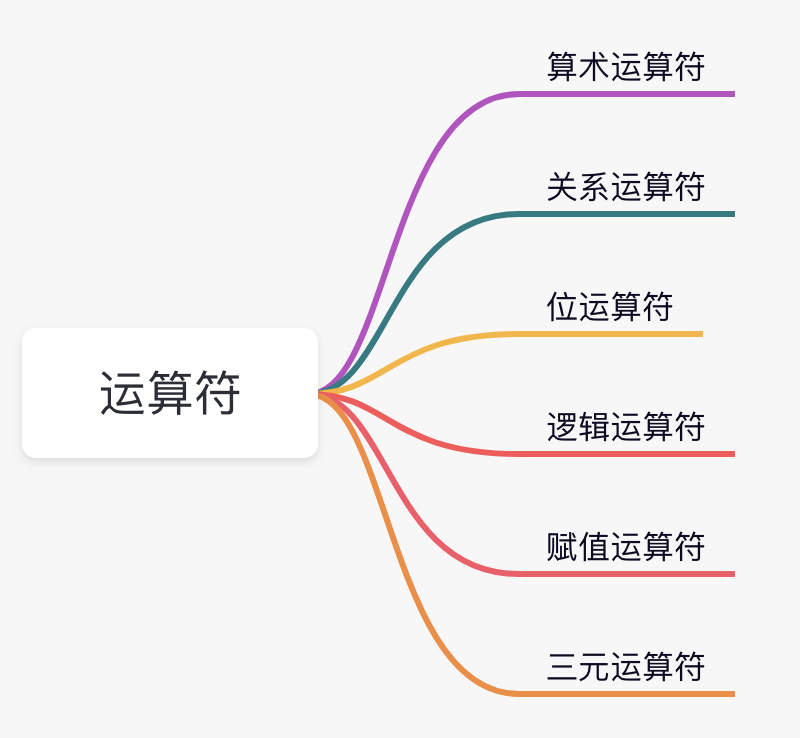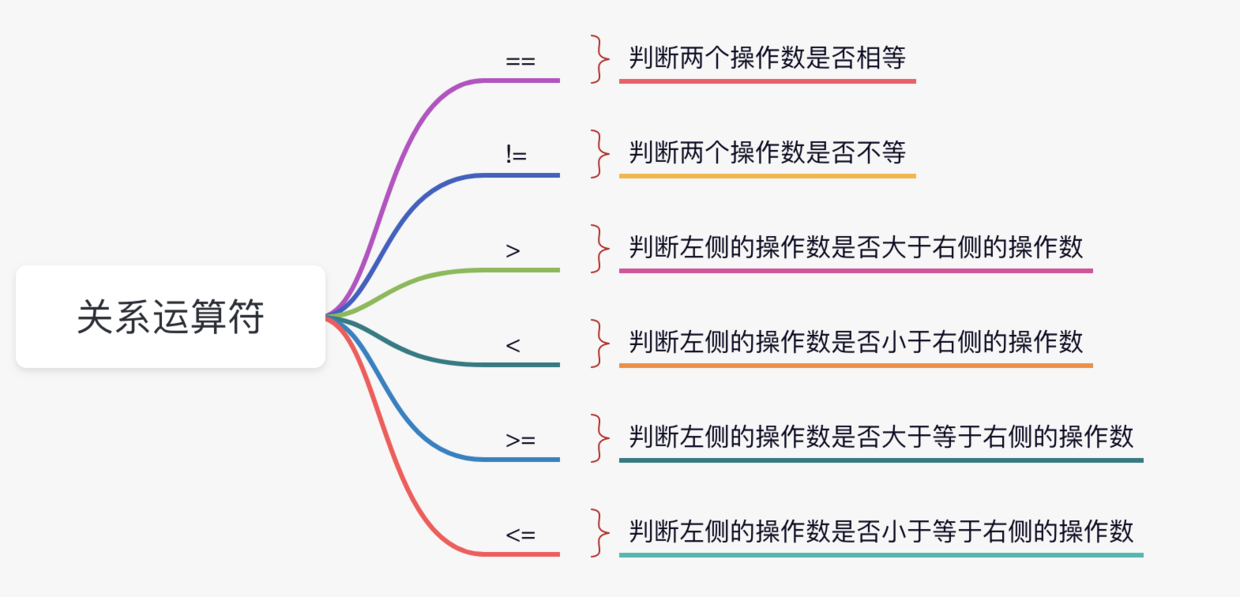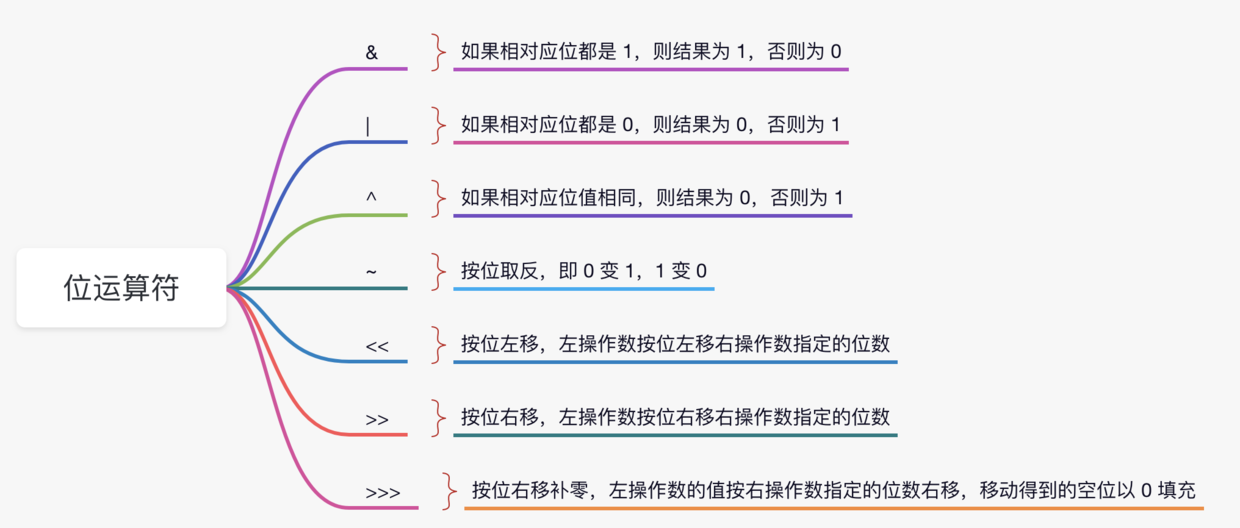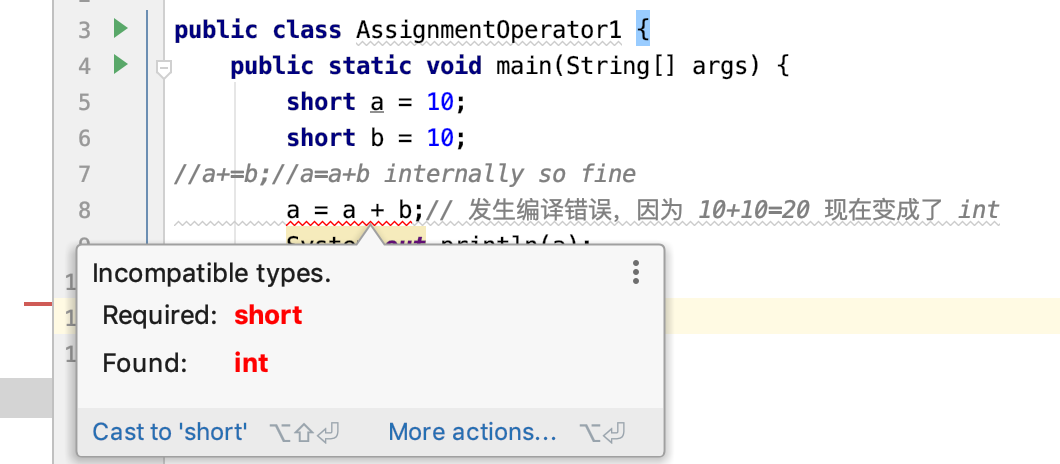# Java 运算符

2021年10月22日

“二哥，让我盲猜一下哈，运算符是不是指的就是加减乘除啊？”三妹的脸上泛着甜甜的笑容，我想她一定对提出的问题很有自信。

“是的，三妹。运算符在 Java 中占据着重要的位置，对程序的执行有着很大的帮助。除了常见的加减乘除，还有许多其他类型的运算符，来看下面这张思维导图。”## # 01、算数运算符

``````/**
* 微信搜索「沉默王二」，回复 Java
*/
public class ArithmeticOperator {
public static void main(String[] args) {
int a = 10;
int b = 5;

System.out.println(a + b);//15
System.out.println(a - b);//5
System.out.println(a * b);//50
System.out.println(a / b);//2
System.out.println(a % b);//0

b = 3;
System.out.println(a + b);//13
System.out.println(a - b);//7
System.out.println(a * b);//30
System.out.println(a / b);//3
System.out.println(a % b);//1
}
}
``````

``````/**
* 微信搜索「沉默王二」，回复 Java
*/
public class ArithmeticOperator {
public static void main(String[] args) {
int a = 10;
float c = 3.0f;
double d = 3.0;
System.out.println(a / c); // 3.3333333
System.out.println(a / d); // 3.3333333333333335
System.out.println(a % c); // 1.0
System.out.println(a % d); // 1.0
}
}
``````

``````System.out.println(10.0 / 0.0); // Infinity
System.out.println(0.0 / 0.0); // NaN
``````

Infinity 的中文意思是无穷大，NaN 的中文意思是这不是一个数字（Not a Number）。

``````Exception in thread "main" java.lang.ArithmeticException: / by zero
at com.itwanger.eleven.ArithmeticOperator.main(ArithmeticOperator.java:32)
``````

``````public class UnaryOperator1 {
public static void main(String[] args) {
int x = 10;
System.out.println(x++);//10 (11)
System.out.println(++x);//12
System.out.println(x--);//12 (11)
System.out.println(--x);//10
}
}
``````

``````int x = 10;
int y = ++x;
System.out.println(y + " " + x);// 11 11

x = 10;
y = x++;
System.out.println(y + " " + x);// 10 11
``````

## # 02、关系运算符``````/**
* 微信搜索「沉默王二」，回复 Java
*/
public class RelationOperator {
public static void main(String[] args) {
int a = 10, b = 20;
System.out.println(a == b); // false
System.out.println(a != b); // true
System.out.println(a > b); // false
System.out.println(a < b); // true
System.out.println(a >= b); // false
System.out.println(a <= b); // true
}
}
``````

## # 03、位运算符

``````/**
* 微信搜索「沉默王二」，回复 Java
*/
public class BitOperator {
public static void main(String[] args) {
System.out.println(Integer.toBinaryString(60)); // 111100
System.out.println(Integer.toBinaryString(13)); // 1101
}
}
``````

PS：现代的二进制记数系统由戈特弗里德·威廉·莱布尼茨于 1679 年设计。莱布尼茨是德意志哲学家、数学家，历史上少见的通才。``````/**
* 微信搜索「沉默王二」，回复 Java
*/
public class BitOperator {
public static void main(String[] args) {
int a = 60, b = 13;
System.out.println("a 的二进制：" + Integer.toBinaryString(a)); // 111100
System.out.println("b 的二进制：" + Integer.toBinaryString(b)); // 1101

int c = a & b;
System.out.println("a & b：" + c + "，二进制是：" + Integer.toBinaryString(c));

c = a | b;
System.out.println("a | b：" + c + "，二进制是：" + Integer.toBinaryString(c));

c = a ^ b;
System.out.println("a ^ b：" + c + "，二进制是：" + Integer.toBinaryString(c));

c = ~a;
System.out.println("~a：" + c + "，二进制是：" + Integer.toBinaryString(c));

c = a << 2;
System.out.println("a << 2：" + c + "，二进制是：" + Integer.toBinaryString(c));

c = a >> 2;
System.out.println("a >> 2：" + c + "，二进制是：" + Integer.toBinaryString(c));

c = a >>> 2;
System.out.println("a >>> 2：" + c + "，二进制是：" + Integer.toBinaryString(c));
}
}
``````

``````static final int hash(Object key) {
int h;
return (key == null) ? 0 : (h = key.hashCode()) ^ (h >>> 16);
}
``````

1）按位左移运算符：

``````public class LeftShiftOperator {
public static void main(String[] args) {
System.out.println(10<<2);//10*2^2=10*4=40
System.out.println(10<<3);//10*2^3=10*8=80
System.out.println(20<<2);//20*2^2=20*4=80
System.out.println(15<<4);//15*2^4=15*16=240
}
}
``````

`10<<2` 等于 10 乘以 2 的 2 次方；`10<<3` 等于 10 乘以 2 的 3 次方。

2）按位右移运算符：

``````public class RightShiftOperator {
public static void main(String[] args) {
System.out.println(10>>2);//10/2^2=10/4=2
System.out.println(20>>2);//20/2^2=20/4=5
System.out.println(20>>3);//20/2^3=20/8=2
}
}
``````

`10>>2` 等于 10 除以 2 的 2 次方；`20>>2` 等于 20 除以 2 的 2 次方。

## # 04、逻辑运算符

``````public class LogicalOperator {
public static void main(String[] args) {
int a=10;
int b=5;
int c=20;
System.out.println(a<b&&a<c);//false && true = false

System.out.println(a>b||a<c);//true || true = true
}
}
``````

``````public class LogicalOperator1 {
public static void main(String[] args) {
int a=10;
int b=5;
int c=20;
System.out.println(a<b&a<c);//false & true = false

System.out.println(a>b|a<c);//true | true = true
}
}
``````

## # 05、赋值运算符

``````public class AssignmentOperator {
public static void main(String[] args) {
int a=10;
int b=20;
a+=4;//a=a+4 (a=10+4)
b-=4;//b=b-4 (b=20-4)
System.out.println(a);
System.out.println(b);
}
}
````````````public class AssignmentOperator1 {
public static void main(String[] args) {
short a = 10;
short b = 10;
//a+=b;//a=a+b internally so fine
a = (short)(a + b);
System.out.println(a);
}
}
``````

``````/**
* 微信搜索「沉默王二」，回复 Java
*/
public class BigIntMulti {
public static void main(String[] args) {
int a = Integer.MAX_VALUE;
int b = 10000;
int c = a * b;
System.out.println(c); // -10000
}
}
``````

``````/**
* 微信搜索「沉默王二」，回复 Java
*/
public class BigIntMulti {
public static void main(String[] args) {
int a = Integer.MAX_VALUE;
int b = 10000;
long c = (long)a * b;
System.out.println(c); // 21474836470000
}
}
``````

``````long a = Integer.MAX_VALUE - 1;
long b = 10000;
long c = a * b;
System.out.println(c); // 21474836460000
``````

## # 06、三元运算符

``````public class TernaryOperator {
public static void main(String[] args) {
int a=2;
int b=5;
int min=(a<b)?a:b;
System.out.println(min);
}
}
``````

“好了，三妹，关于 Java 运算符就先说这么多吧，你是不是已经清楚了？”转动了一下僵硬的脖子后，我对三妹说。

“差不多，二哥，我需要写点 demo 练习会。”